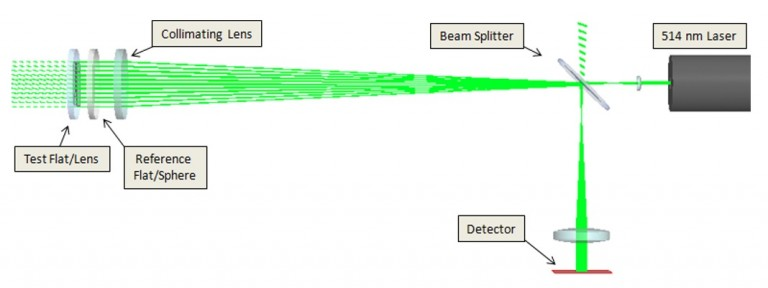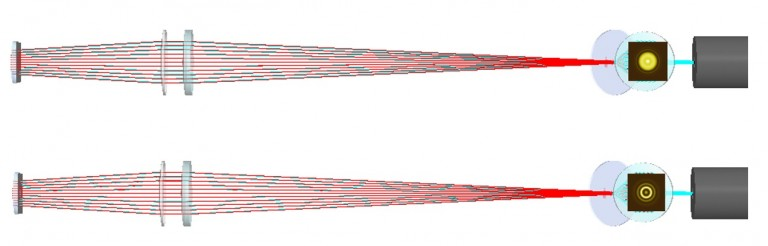# Fizeau Measurement of a Lens Surface

## Introduction

FRED allows for simulation of physical optics phenomena such as diffraction and interference. With this capability, components such as Gaussian laser beams and interferometers can be accurately modeled and incorporated into optical systems.

## The FRED Model

Interferometers can be used in metrology to measure surface quality of manufactured lenses and mirrors. A Fizeau interferometer is commonly used for such a measurement (Figure 1).Figure 1Fizeau interferometer for surface measuremetns. Laser light is expanded and collimated. Interference occurs between reflections from a reference optic and test optic. If the test opic is a lens or curved mirror, a reference sphere is used to produce a converging wavefront. The test surface is placed in its matching wavefront, and defects are measured relative to this wavefront.

To modify the FRED file in Figure 1 for lens metrology, the reference flat becomes a reference sphere and the test flat becomes a plano-convex lens. The reference flat has a back radius of -70. Its curved surface is transmissive and its flat surface is uncoated. The test flat a front radius of 80. It is relocated to a Z shift of 54.25. The imaging lens has a Z-shift of 150 and the detector has a Z-shift of 10. The test lens is located in its nominal wavefront, and a slight axial displacement of this lens leads to interference fringes (Figure 2).Figure 2Fizeau measurement of lens surface. When the lens is in its nominal wavefront, a null interferogrm is formed. If the lens is axially displaced (in this case, brought closer with a Z shift of 53), power fringes appear in the interferogram.